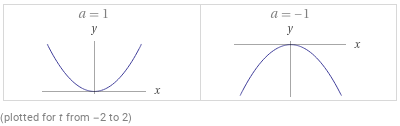Search IntMath
Close

450+ Math Lessons written by Math Professors and Teachers

5 Million+ Students Helped Each Year

1200+ Articles Written by Math Educators and Enthusiasts

Simplifying and Teaching Math for Over 23 Years

# Exploring the Eccentricity of Parabolas in Geometry## What is a Parabola?

A parabola is a conic section in the shape of an open, curved U-shape. It is a type of symmetrical curve that can be seen in many areas of mathematics, including geometry and calculus. In geometry, a parabola is a two-dimensional curve that can be described by its equation, which is usually written in the form of a quadratic equation. Parabolas are part of the family of conic sections, which includes circles, ellipses, and hyperbolas.

Parabolas can be used to model many real-world phenomena, such as trajectories of projectiles. They are also widely used in engineering, architecture, and art. Parabolas can be found in nature, too. Examples include the shape of some leaves and the pattern of a sunflower�s seeds.

## What is Eccentricity?

In geometry, eccentricity is a measure of how closely a parabola resembles a circle. A perfectly circular parabola has an eccentricity of zero, while an extremely elongated parabola has an eccentricity of one. Eccentricity is used to describe the shape of a parabola, and is useful for describing the properties of a parabola.

Eccentricity can be calculated by taking the ratio of the distance between two foci of the parabola (called the �focal length�) to the length of the parabola�s longest chord (called the �directrix�). The formula for eccentricity is e = focal length/directrix. If the focal length is equal to the directrix, the eccentricity is zero, and the parabola is perfectly symmetrical. If the focal length is greater than the directrix, the eccentricity is greater than zero, and the parabola is elongated.

## Using Eccentricity to Describe a Parabola

The eccentricity of a parabola is an important tool for describing its shape. It can be used to determine the orientation of the parabola (whether it is horizontal, vertical, or somewhere in between). It can also be used to determine the size and shape of the parabola, as well as its focus and directrix. It is also useful for predicting the behavior of a parabola in various situations.

For example, if a parabola has an eccentricity of zero, it is perfectly symmetrical. This means that it is equally curved in all directions, and its focus is located at its center. On the other hand, if a parabola has an eccentricity greater than zero, it is elongated in one direction and its focus is located on one side of the parabola. Knowing the eccentricity of a parabola can help you predict its behavior in various situations.

## Practice Problems

1. Find the eccentricity of a parabola with a focal length of 5 and a directrix of 10.

Answer: The eccentricity of the parabola is 0.5.

2. Find the focal length and directrix of a parabola with an eccentricity of 0.3.

Answer: The focal length of the parabola is 3 and the directrix is 10.

3. Find the orientation of a parabola with an eccentricity of 0.6.

Answer: The parabola is elongated in one direction and its focus is located on one side of the parabola.

4. Find the equation of a parabola with a focus at (2, 1) and a directrix of y = -2.

Answer: The equation of the parabola is (x - 2)^2 = -4(y - 1).

5. Find the size and shape of a parabola with a focal length of 4 and a directrix of 8.

Answer: The size of the parabola is 4 and the shape is elongated in one direction with the focus located on one side of the parabola.

6. Find the focus and directrix of a parabola with an eccentricity of 0.7.

Answer: The focus of the parabola is 7 and the directrix is 10.

## Conclusion

In conclusion, eccentricity is an important tool for describing the shape of a parabola. It is useful for predicting the behavior of a parabola in various situations, as well as determining the size and shape of the parabola, and its focus and directrix. Understanding eccentricity can help you better understand parabolas and their properties.

## FAQ

### What is the eccentricity of a parabola?

The eccentricity of a parabola is equal to 1.

### How does eccentricity affect the shape of a parabola?

The eccentricity of a parabola affects its shape by determining the height of the vertex in relation to the length of the focus. The eccentricity of a parabola is always 1, so the vertex is always located at the same distance from the focus regardless of the shape of the parabola.

## Problem SolverThis tool combines the power of mathematical computation engine that excels at solving mathematical formulas with the power of GPT large language models to parse and generate natural language. This creates math problem solver thats more accurate than ChatGPT, more flexible than a calculator, and faster answers than a human tutor. Learn More.